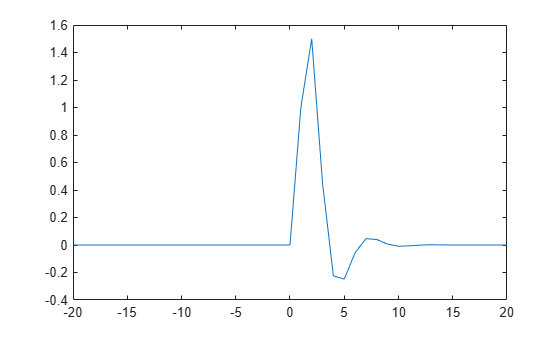# kroneckerDelta

Kronecker delta function

## Syntax

``kroneckerDelta(m)``
``kroneckerDelta(m,n)``

## Description

example

````kroneckerDelta(m)` returns `1` if `m == 0` and `0` if `m ~= 0`.```

example

````kroneckerDelta(m,n)` returns `1` if `m == n` and `0` if `m ~= n`.```

## Examples

### Compare Two Symbolic Variables

Note

For `kroneckerDelta` with numeric inputs, use the `eq` function instead.

Set symbolic variable `m` equal to symbolic variable `n` and test their equality using `kroneckerDelta`.

```syms m n m = n; kroneckerDelta(m,n)```
```ans = 1```

`kroneckerDelta` returns `1` indicating that the inputs are equal.

Compare symbolic variables `p` and `q`.

```syms p q kroneckerDelta(p,q)```
```ans = kroneckerDelta(p - q, 0)```

`kroneckerDelta` cannot decide if `p == q` and returns the function call with the undecidable input. Note that `kroneckerDelta(p, q)` is equal to ```kroneckerDelta(p - q, 0)```.

To force a logical result for undecidable inputs, use `isAlways`. The `isAlways` function issues a warning and returns logical `0` (`false`) for undecidable inputs. Set the `Unknown` option to `false` to suppress the warning.

`isAlways(kroneckerDelta(p, q), 'Unknown', 'false')`
```ans = logical 0```

### Compare Symbolic Variable with Zero

Set symbolic variable `m` to `0` and test `m` for equality with `0`. The `kroneckerDelta` function errors because it does not accept numeric inputs of type `double`.

```m = 0; kroneckerDelta(m)```
`Undefined function 'kroneckerDelta' for input arguments of type 'double'.`

Use `sym` to convert `0` to a symbolic object before assigning it to `m`. This is because `kroneckerDelta` only accepts symbolic inputs.

```syms m m = sym(0); kroneckerDelta(m)```
```ans = 1```

`kroneckerDelta` returns `1` indicating that `m` is equal to `0`. Note that `kroneckerDelta(m)` is equal to ```kroneckerDelta(m, 0)```.

### Compare Vector of Numbers with Symbolic Variable

Compare a vector of numbers `[1 2 3 4]` with symbolic variable `m`. Set `m` to `3`.

```V = 1:4 syms m m = sym(3) sol = kroneckerDelta(V,m)```
```V = 1 2 3 4 m = 3 sol = [ 0, 0, 1, 0]```

`kroneckerDelta` acts on `V` element-wise to return a vector, `sol`, which is the same size as `V`. The third element of `sol` is `1` indicating that the third element of `V` equals `m`.

### Compare Two Matrices

Compare matrices `A` and `B`.

Declare matrices `A` and `B`.

```syms m A = [m m+1 m+2;m-2 m-1 m] B = [m m+3 m+2;m-1 m-1 m+1]```
```A = [ m, m + 1, m + 2] [ m - 2, m - 1, m] B = [ m, m + 3, m + 2] [ m - 1, m - 1, m + 1]```

Compare `A` and `B` using `kroneckerDelta`.

`sol = kroneckerDelta(A,B)`
```sol = [ 1, 0, 1] [ 0, 1, 0]```

`kroneckerDelta` acts on `A` and `B` element-wise to return the matrix `sol` which is the same size as `A` and `B`. The elements of `sol` that are `1` indicate that the corresponding elements of `A` and `B` are equal. The elements of `sol` that are `0` indicate that the corresponding elements of `A` and `B` are not equal.

### Use `kroneckerDelta` in Inputs to Other Functions

`kroneckerDelta` appears in the output of `iztrans`.

```syms z n sol = iztrans(1/(z-1), z, n)```
```sol = 1 - kroneckerDelta(n, 0)```

Use this output as input to `ztrans` to return the initial input expression.

`ztrans(sol, n, z)`
```ans = z/(z - 1) - 1```

### Filter Response to Kronecker Delta Input

Use `filter` to find the response of a filter when the input is the Kronecker Delta function. Convert `k` to a symbolic vector using `sym` because `kroneckerDelta` only accepts symbolic inputs, and convert it back to double using `double`. Provide arbitrary filter coefficients `a` and `b` for simplicity.

```b = [0 1 1]; a = [1 -0.5 0.3]; k = -20:20; x = double(kroneckerDelta(sym(k))); y = filter(b,a,x); plot(k,y)```## Input Arguments

collapse all

Input, specified as a number, vector, matrix, multidimensional array, or a symbolic number, vector, matrix, function, or multidimensional array. At least one of the inputs, `m` or `n`, must be symbolic.

Input, specified as a number, vector, matrix, multidimensional array, or a symbolic number, vector, matrix, function, or multidimensional array. At least one of the inputs, `m` or `n`, must be symbolic.

collapse all

### Kronecker Delta Function

The Kronecker delta function is defined as

## Version History

Introduced in R2014b